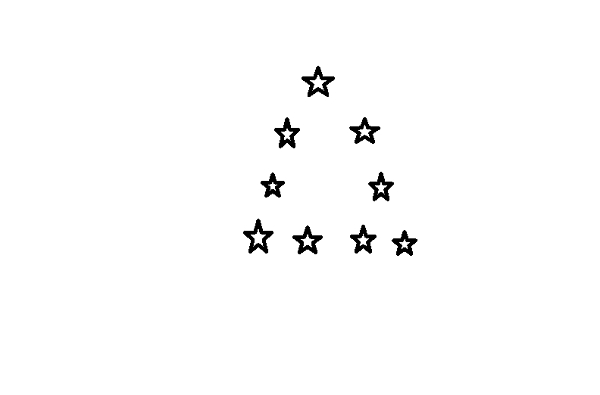# Finding whether a number is triangular number in JavaScript

## Triangular Number

Triangular number is the number of points that can fill an equilateral triangle.

For instance − 9 is a triangular number which makes an equilateral triangle with each side of 4 units.## Problem

We are required to write a JavaScript function that takes in a number and returns true if its a triangular number, false otherwise.

## Example

Following is the code −

Live Demo

const num = 9;
const isTriangular = (num = 1) => {
let i = 4;
if(num === 1){
return true;
};
if(num === 3){
return true;
};
while(((3 * 1) - 3) <= num){
if((3 * i) - 3 === num){
return true;
};
i++;
}
return false;
};
console.log(isTriangular(num));

## Output

Following is the console output −

true